# How to Use SUMPRODUCT to Calculate Weighted Averages in MS Excel 2013

## Weighted Averages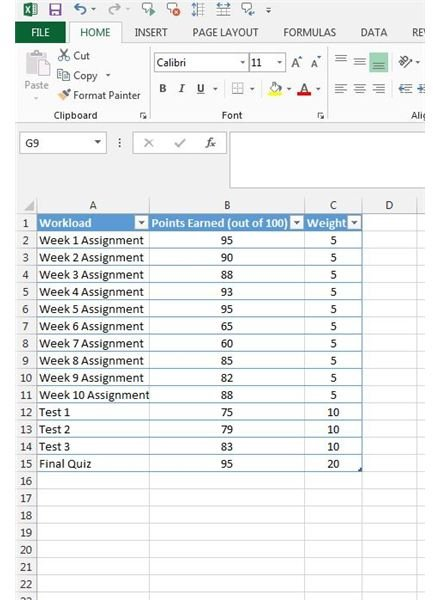The easiest way to visualize weighted averages is to picture yourself back in school. As you may remember, some assignments would represent a larger portion of your grade than others would. For instance, a final exam may represent a large chunk of your grade for the class, whereas a weekly assignment may only be a small fraction of the final grade.

I have set up a spreadsheet with some sample data showing various work assignments, the points assigned and the weight for the assignment (Figure 1). If we simply determined the average grade earned, it would be 83.8. The weighted average however will be 84.8. Not a huge difference in this case, but the distinction is important, especially if we assign a larger weight to some of the assignments. Let’s go through how to figure out the weighted average.

## Finding the Weighted Average

There are a number of ways to find the weighted average. The method you choose depends on how visual you are. Using my sample spreadsheet, if you only want the formula, here it is: SUMPRODUCT(B2:B15, C2:C15)/SUM(C2:C15).

## Images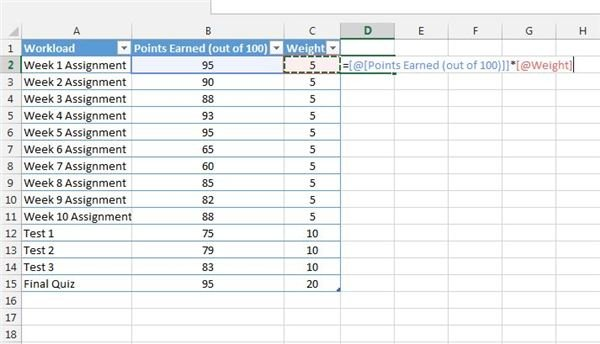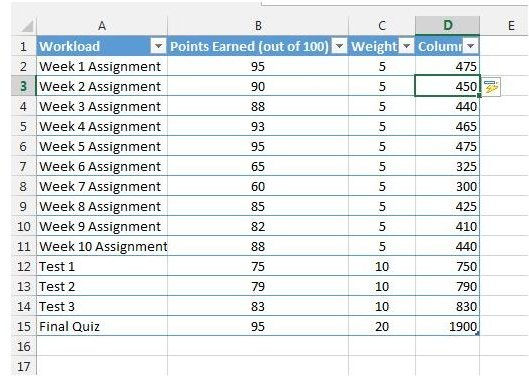## Weighted Average

So what’s happening here? The SUMPRODUCT function performs two operations. First, it takes the product (aka multiplies) one cell by another cell. Then it adds up the resulting values. Thus the name SUMPRODUCT.

If we were to do this the long way, we would use Column D to hold the product of Column B and Column C. We can do this by typing “=” into the formula bar for cell D2, selecting B2, pressing the “*” key and then selecting cell C2. You should see the formula as shown in Figure 2. When you press Enter, Excel 2013 is smart enough to know you want to find the product of column B and C for your table, so it should fill in all of the remaining cells in column D (Figure 3).

Next, we need to add up Column D. In cell D16 type the =SUM(and select cells D2 through D15. Hit Enter. You should now have your “SUMPRODUCT”.

## Images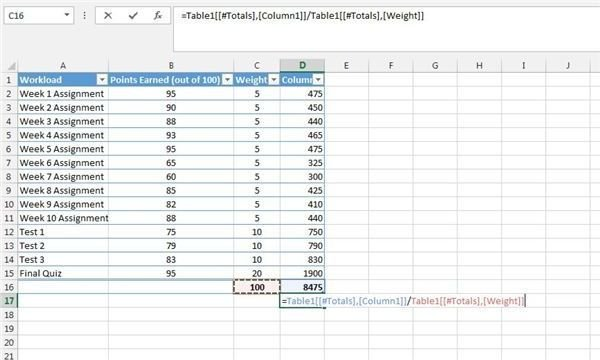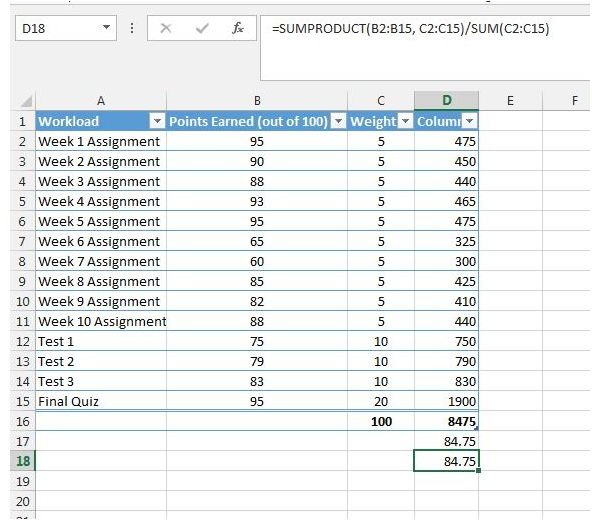## Weighted Average

The second part of the formula is to take your SUMPRODUCT and divide it by the sum of the weights. In my case, the sum of my weights is 100. It doesn’t have to be 100 – your weights can be whatever you want them to be. As we did for Cell D16, put “=SUM(” in C16 and select cells C2 through C15. Hit Enter.

You should have the sum of your weights in cell C16 and the sum of your products of columns B and C in cell D16. Now we can divide our SUMPRODUCT/SUM(ofWeights). Place your cursor wherever you want your final weighted average to be and type “=”. Select cell D16. Type “/” and then select cell C16 (Figure 4). Hit Enter and there you go – you have your weighted average.

You will notice I have the same result when using the SUMPRODUCT formula in Figure 5.